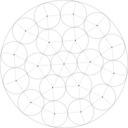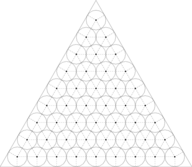New and Improved Results in the packing of identical unitary radius circles

## Introduction

The problem at hand is that of packing a set of N circles (hereinafter called items) of unitary radius in a fixed-shaped figure (denominated object) while minimizing the latter's dimensions. Throughout this work, several geometric forms were treated, viz. circle, square, rectangle, triangle and strip, which is defined as a rectangle that has got one of its dimensions fixed.

This page shows numerical results and visual solutions for the problems considered in:

E. G. Birgin and J. M. Gentil, "New and improved results for packing identical unitary radius circles within triangles, rectangles and strips", Computers & Operations Research 37, pp. 1318-1327, 2010. [Abstract] [pdf]

## Unitary radius circles

The objects considered here are: circle, square, strip, equilateral triangle and rectangle.

 Packing of unitary-radius circles in a circle Numerical results and graphics [source] Packing of unitary-radius circles in a rectangle (minimizing area) Numerical results and graphics [source] Packing of unitary-radius circles in a rectangle (minimizing perimeter) Numerical results and graphics [source] Packing of unitary-radius circles in a square Numerical results and graphics [source] Packing of unitary-radius circles in a strip Numerical results and graphics [source] Packing of unitary-radius circles in an equilateral triangle Numerical results and graphics [source]

### Description of files

The .dat files contain all the information about the desired problem and the coordinates of the circles for the final packing, which includes all the information in the tables and the solution coordinates for each circle.

The .mp files are MetaPost files. To visualize the solutions, just follow the steps below. At a command line, type```mp desired-file.mp
```

A file named desired-file.1 (an EPS file) will be created. Rename it to figure.1. Now you have to include this recently created file into a TeX file. Download the file figures.tex. Then type

```latex figures.tex
dvips figures.dvi -o figures.ps
```

Now type

```gv figures.ps
```

to see the desired solution.